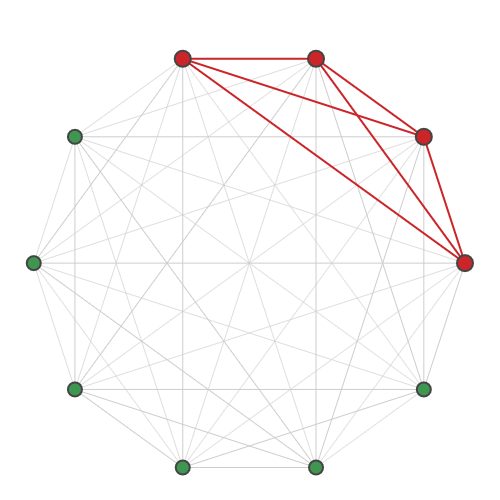# sf.apps.plot.graph¶

graph(g, s=None, plot_size=(500, 500))[source]

Creates a plot of the input graph.

This function can plot the input graph only, or the graph with a specified subgraph highlighted. Graphs are plotted using the Kamada-Kawai layout with an aspect ratio of 1:1.

Example usage:

>>> graph = nx.complete_graph(10)
>>> fig = plot.graph(graph, [0, 1, 2, 3])
>>> fig.show()Parameters
• g (nx.Graph) – input graph

• s (list) – optional list of nodes comprising the subgraph to highlight

• plot_size (int) – size of the plot in pixels, given as a pair of integers (x_size, y_size)

Returns

figure for graph and optionally highlighted subgraph

Return type

Figure

### Contents

Using Strawberry Fields

Development

API